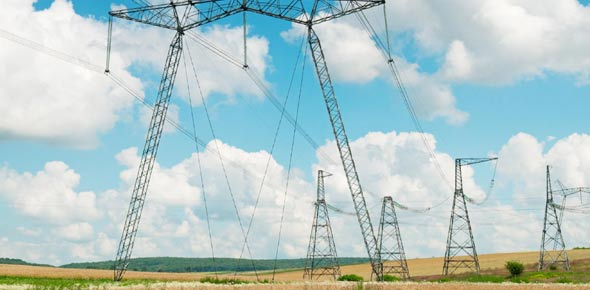# Electric Charges And Electricity MCQ: Quiz

10 Questions | Attempts: 363
ShareSettingsHow Much Do You Know About Electric Charges And Electricity? Electricity is all around us, and it is used in almost every building. There are different electric current sources, and the quiz below is perfect for seeing just how much you know about electricity. How about you give it a shot and get to see how well you will do!

• 1.
Define as the flow of electrons or electric current. It is an invisible form of energy that can be transformed into another form of energy like heat light and mechanical.
• A.

Electricity

• B.

Resistance

• C.

Proton

• D.

Electron

• 2.
The negative charge component of an atom. It has the ability to flow.
• A.

Electricity

• B.

Resistance

• C.

Proton

• D.

Electron

• 3.
The positive charge component of an atom. It had the ability to attract an electron.
• A.

Electricity

• B.

Resistance

• C.

Proton

• D.

Electron

• 4.
Anything that occupies space and has mass. It can be a solid, liquid, or gas in the state.
• A.

Atom

• B.

Matter

• C.

Current

• D.

Voltage

• 5.
The amount of pulling force that makes the electron flow. Also known as potential difference or EMF(electromotive force). The amount of voltage can be measured in volt (V).
• A.

Atom

• B.

Matter

• C.

Current

• D.

Voltage

• 6.
The flow of electric charge is only in one direction.
• A.

AC

• B.

DC

• C.

BC

• D.

CC

• 7.
The movement of electric charge periodically reverses direction.
• A.

AC

• B.

DC

• C.

BC

• D.

DC

• 8.
Commonly represented by a bulb or any electrical component that consumes electricity.
• A.

Electricity

• B.

Supply

• C.

Switch

• D.

• 9.
Type of circuit that has an open path for current to flow. It means that the current cannot flow in an open circuit.
• A.

• B.

Supply

• C.

Open

• D.

Closed

• 10.
Materials that easily allow the flow of current.
• A.

Insulator

• B.

Conductor

• C.

AC

• D.

DC

## Related TopicsBack to top
×

Wait!
Here's an interesting quiz for you.